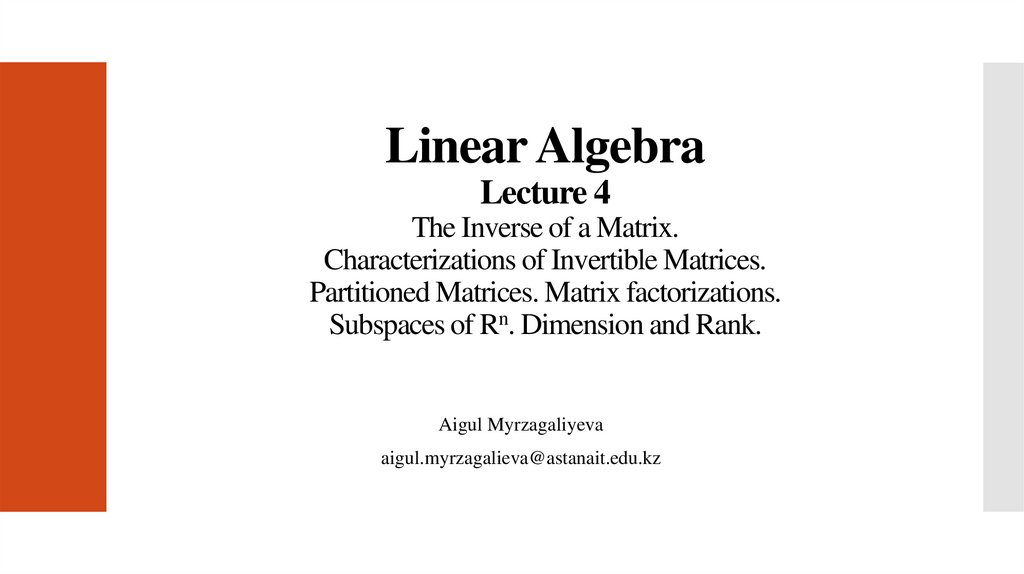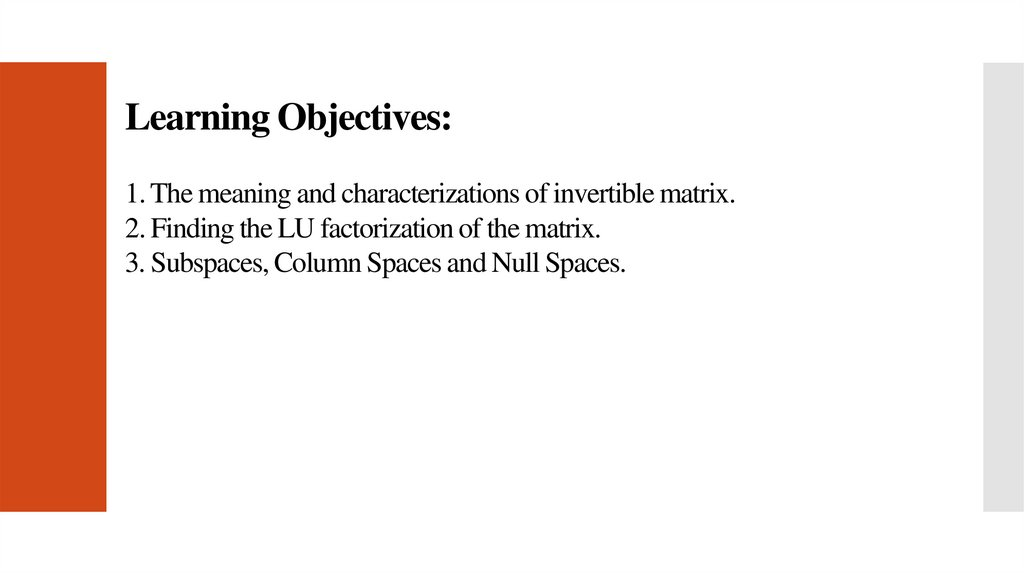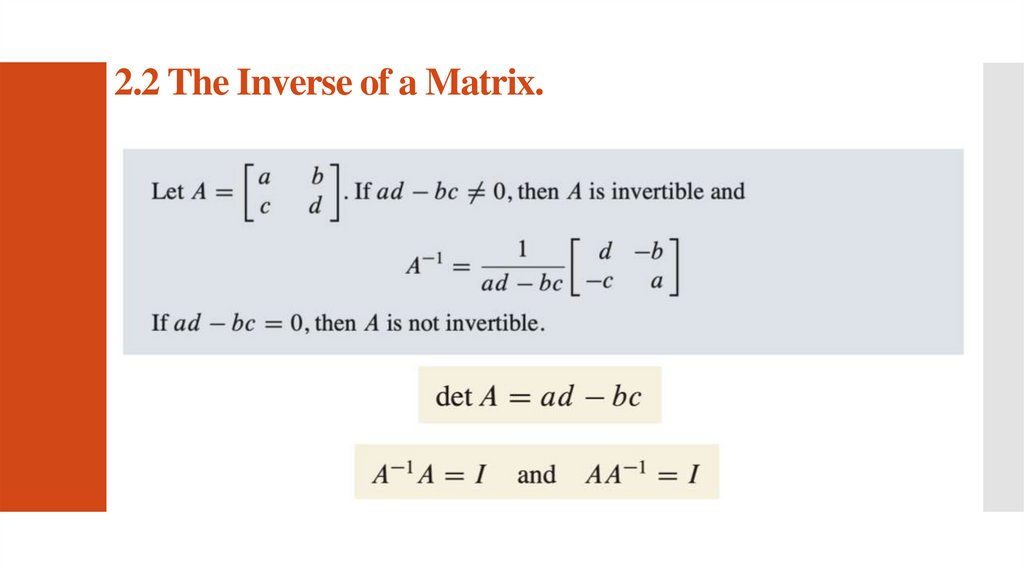# Linear Algebra. Lecture 4

## 1.

Linear Algebra
Lecture 4
The Inverse of a Matrix.
Characterizations of Invertible Matrices.
Partitioned Matrices. Matrix factorizations.
Subspaces of Rn. Dimension and Rank.
Aigul Myrzagaliyeva
[email protected]

## 2.

Learning Objectives:
1. The meaning and characterizations of invertible matrix.
2. Finding the LU factorization of the matrix.
3. Subspaces, Column Spaces and Null Spaces.

## 3.

2.2 The Inverse of a Matrix.

## 6.

An Algorithm for Finding A-1

## 7.

2.3. Characterizations of Invertible Matrices.

## 9.

2.4. Partitioned Matrices.
A block matrix or a partitioned matrix is a partition of a matrix into rectangular
smaller matrices called blocks.

## 11.

2.5. Matrix Factorizations.
A factorization of a matrix A is an equation that expresses A as a product of two
or more matrices.
The LU Factorization
Ly=b
Ux=y

1)
2)

## 13.

2.8. Subspaces of Rn.

## 15.

Column Space and Null Space of a Matrix

## 17.

2.9. Dimension and Rank.